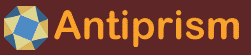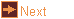# iso_kite - isohedral kite-faced polyhedra

 Usage    |    Examples    |    Notes

## Usage

```

Usage: iso_kite [options] model_args...

Create all isohedral polyhedra and polyhedron compounds whose face type
is a kite (or dart)

Model_args can be a Schwarz triangle name (which can also be given as two
or three fractions A, B, C (default 2) which specify the Schwarz triangle
vertices, where n/d corresponds to an angle PI*d/n), optionally followed by
a permutation letter from AaBbCc, to rotate the fraction A, B, or C to the
front (and if lowercase then follow by swapping the last two fractions)
T1:  (3 3 2)         T2:  (3 3 3/2)
O1:  (4 3 2)         O2:  (4/3 4 3)
I1:  (5 3 2)         I2:  (5/2 3 2)
I3:  (5/2 5 2)       I4:  (5/2 3 3)
I5:  (5/4 3 3)       I6:  (5/3 5 3)
I7:  (5/4 5 3)       I8:  (5/3 5/2 3)
I9:  (5/4 5 5)       I10: (5/2 5/2 5/2)
If model_args is a single fraction then make a trapezohedron (with B and C
as antiprism vertices).
The kite has vertices along A, C, B, and C reflected in AB. The height of up
to two vertices may be given, and the third is calculated (B and C have
the same height in a trapezohedron). If less than two heights are given
the calculated vertex will be B, if not specified, otherwise A. The kite
is repeated with the symmetry corresponding to the base Schwarz triangle,
or trapezohedron model.
Notes: the tetrahedral triangles T1 and T2 both produce a model with
octahedral symmetry when height A=-B (cube and rhombic dodecahedron,
respectively). These models do not form an octahedral compound, and have
common symmetry subgroup of Th in the icosahedral compounds. The 3
trapezohedron also has octahedral symmetry at height A=+/-B (cube), and
likewise has a common symmetry subgroup of Th in icosahedral compounds.

Options
-h,--help this help message (run 'off_util -H help' for general help)
--version version information
-l num    'list' will list all compounds of the specified base model, or
all models and compounds if no base model specified. The list
includes a model number and base model, and if a compound, the
alignment symmetry (possibly with realignment number) and final
symmetry (and possibly a final pre-realignment number).
Specifying a number will make the the model with that number
in the list.
-A ht     height of kite apex on OA (default 1.0 or calculated)
-B ht     height of kite apex on OB (only magnitude used if trapezohedron,
default 1.0 or calculated)
-C ht     height of kite side vertex on OC (Schwarz triangle only,
default 1.0 or calculated)
-a ang    angle, for compounds where this is a parameter
-N parts  number of parts, for compounds where this is a parameter
-c type   colour the faces around each vertex of a type, from AaBbCc
(colouring by value/index for upper/lower case) using a
different colour for each set (Schwarz models only), or Kk
to colour by base component (which may be compound)
-k        output a single kite (colours not applied)
-q        quiet, don't print final report
-o <file> write output to file (default: write to standard output)

```

## Examples

Specify a trapezohedron by its polygon fraction
```   iso_kite 5 | antiview
iso_kite 3/2 | antiview
```

General member of series including deltoidal hexacontahedron, can be specified as 3 fractions, 2 fractions (the third defaults to 2) or by the triangle name (see iso_kite -h for the list of names).
```   iso_kite 5 3 2 | antiview
iso_kite 5 3 | antiview
iso_kite I1 | antiview
```

Get a list of all models, all models involving a 3 trapezohedron (parallelopiped), all models involving the (3 3 2) triangle series
```   iso_kite
iso_kite -l list 3
iso_kite -l list 3 3
```

Make the second model in each of these lists
```   iso_kite -l 2 | antiview
iso_kite -l 2 3 | antiview
iso_kite -l 2 3 3 | antiview
```

General member of series including deltoidal icositetrahedron. Set a height for the point along the first vertex
```   iso_kite -A 2.5 3 4 | antiview
```

General member of series including deltoidal icositetrahedron. The vertex order is significant
```   iso_kite -A 2.5 4 3 | antiview
```

Set a height for the C vertex, which is one of the kite's side vertices.
```   iso_kite -C 2.5 3 4 | antiview
```

Set any two heights of a Schwarz triangle model or both A and B for a trapezohedron (although some height combinations will produce a degenerate or non-constructible model).
```   iso_kite -A 1 -B 2 3 4 | antiview
iso_kite -A 1 -C 2 3 4 | antiview
iso_kite -B 2 -C 2 3 4 | antiview
iso_kite -A 1 -B 1 3 | antiview
```

Negative heights are allowed, or instead of using a negative height for, say, A, the B and C fractions could be replaced with their supplements
```   iso_kite -A -2.5 5 3 2 | antiview
iso_kite -A 2.5 5 3/2 2 | antiview
```

Colour faces around vertices of type A (the first vertex, in this case with angle 4) in sets
```   iso_kite -c A -A 2.5 4 3 | antiview
```

Colour faces around vertices of type B (the second vertex, in this case with angle 3) in sets
```   iso_kite -c B -A 2.5 4 3 | antiview
```

Colour faces around vertices of type C (the third vertex, in this case with angle 3). With vertex C only half the faces around each vertex have the same colour
```   iso_kite -c C -A 2.5 4 3 | antiview
```

Colour by component
```   iso_kite -c K -A 3 3 -l 5 | antiview
```

For some trapezohedron compounds, the colouring component is a pair of trapezohedra
```   iso_kite -c K -A 3 3 -l 6 | antiview
```

The dual models can be attractive
```   iso_kite -A 0.2 5/4 5/3 | pol_recip | antiview
```

Models with A or B as 2 will be degenerate, and have triangles for kites. These, and other, degenerate models cannot be used with the -l listing option
```   iso_kite 3 2 4| antiview
```

## Notes

See the Wikipedia page on Schwarz triangles for a description and list of triangles.